## NAME

r.boxplot - Draws the boxplot of raster values. Optionally, this is done per category of a zonal raster layer

## KEYWORDS

display, raster, plot, boxplot

## SYNOPSIS

r.boxplot
r.boxplot --help
r.boxplot [-onhsc] input=name [zones=name] [output=name] [plot_dimensions=string] [fontsize=integer] [dpi=integer] [map_outliers=string] [range=float] [raster_statistics=string[,string,...]] [bx_sort=string] [rotate_labels=float] [raster_stat_color=name] [raster_stat_alpha=float] [bx_color=name] [bx_width=float] [bx_lw=float] [median_lw=float] [median_color=name] [whisker_linewidth=float] [flier_marker=string] [flier_size=string] [flier_color=name] [--overwrite] [--help] [--verbose] [--quiet] [--ui]

### Flags:

-o
Include outliers
Draw boxplot(s) with outliers
-n
Draw notches
Draw boxplot(s) with notch
-h
Horizontal boxplot(s)
Draw the boxplot horizontal
-s
Show category numbers
Show the category numbers of the zonal map
-c
Zonal colors
Color boxploxs using the colors of the categories of the zonal raster
--overwrite
Allow output files to overwrite existing files
--help
Print usage summary
--verbose
Verbose module output
--quiet
Quiet module output
--ui
Force launching GUI dialog

### Parameters:

input=name [required]
Input raster
input raster
zones=name
Zonal raster
categorical map with zones
output=name
Name of output image file
Name for output file
plot_dimensions=string
Plot dimensions (width,height)
Dimensions (width,height) of the figure in inches
fontsize=integer
Font size
Default font size
Default: 10
dpi=integer
DPI
resolution of plot
map_outliers=string
Name of outlier map
Create a vector point layer of outliers
range=float
Range (value > 0)
this determines how far the plot whiskers extend out from the box. If range is positive, the whiskers extend to the most extreme data point which is no more than range times the interquartile range from the box. A value of zero causes the whiskers to extend to the data extremes.
Default: 1.5
raster_statistics=string[,string,...]
Plot the raster median and IQR
Options: median, IQR
bx_sort=string
Sort boxplots
Sort boxplots based on their median values
Options: descending, ascending
rotate_labels=float
Rotate labels
Rotate labels (degrees)
Options: -90-90
raster_stat_color=name
Color of the raster IQR and median
Color of raster IQR and median
Default: grey
raster_stat_alpha=float
Transparency of the raster IQR band
Options: 0-1
Default: 0.2
bx_color=name
Color of the boxplots
Color of boxplots
Default: white
bx_width=float
Boxplot width
The width of the boxplots (0,1])
Options: 0-1
Default: 0.75
bx_lw=float
boxplot linewidth
The linewidth of the boxplots
Default: 1
median_lw=float
width of the boxplot median line
Default: 1.1
median_color=name
Color of the boxlot median line
Color of median
Default: orange
whisker_linewidth=float
Whisker and cap linewidth
The linewidth of the whiskers and caps
Default: 1
flier_marker=string
Flier marker
Set flier marker (see https://matplotlib.org/stable/api/markers_api.html for options)
Default: o
flier_size=string
Flier size
Set the flier size
Default: 2
flier_color=name
Flier color
Set the flier color
Default: black

## DESCRIPTION

r.boxplot draws boxplots of the raster values of an input raster layer. The user has the option to define a zonal (categorical) layer. In that case, a boxplot will be drawn for each zone, using the values of the input raster that fall within that zone.

If there is a zonal map, the user can add a line and band to represent the median and interquartile range of the input layer. Note that all values of the input raster (within the region's extent) are used to compute the median and IQR. If the zones of your zonal map cover only part of the region, you can use r.mask to mask out the non-covered parts of the input map first. That will result in an IQR and median representing the values that fall within the zones of the zonal map only.

By default, the resulting plot is displayed on screen. However, the user can also save the plot to file using the output option. The format is determined by the extension given by the user. So, if output = outputfile.png, the plot will be saved as a png file.

The whiskers extend to the most extreme data point, which is no more than range ✕ the interquartile range (iqr) from the box. By default, a range of 1.5 is used, but the user can change this. Note that range values need to be larger than 0.

By default, outliers are not included in the plot. Set the -o flag to include them in the plot. To also create a point vector map with the locations of the outliers, the user needs to provide the name of the output map using map_outliers.

There are a few layout options, including the option to rotate the plot and the x-axis labels, print the boxplot(s) with notches, sort the boxplot from low to high (ascending) or from high to low (descending) median, color the boxplots according to the corresponding categories of the zonal raster, set the type and color of the outliers, set the color and width of the median line(s), set the color and transparency of the raster's median line and IQR band, and set the width of the boxplots.

## NOTE

The r.boxplot module operates on the raster array defined by the current region settings, not the original extent and resolution of the input map. See g.region to understand the impact of the region settings on the calculations. To include outliers, the function converts the raster cell with outlier values to a point vector layer. This may take some time if there are a lot of outliers. So, if you are working with very large raster layers, be cautious to not set the range value very low as that may result in a huge number of outliers.

The zonal map needs to be an integer map. If it is not, the function will exit with the error message, 'The zonal raster must be of type CELL (integer)'.

If the -c flag is used, the bxp_color and median_color are ignored, even if set by the user. The option to color boxploxs using the colors of the categories of the zonal raster (-c flag) only works if the zonal map contains a color table. If it does not, the function exits with the error message that 'The zonal map does not have a color table'. If you think there is a color table, run r.colors.out and check if the categories are integers. If not, that is the problem. If they are all integers, you probably have caught a bug.

The module respects the mask (if set), and the region settings. This means you can quickly change the area for which to create a boxplot by simply changing the region and/or setting a (different) mask.

## EXAMPLE

### Example 1

Draw a boxplot of the values of the elevation layer from the NC sample dataset. Set the -h flag to print the boxplot horizontally. Set the plot dimensions to 7 inch wide, 1 inch high.
```g.region raster=elevation
r.boxplot -h input=elevation plot_dimensions="7,1" output="r_boxplot_01.png"
```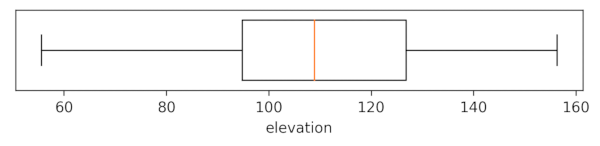### Example 2

Draw boxplots of the values of the elevation layer per category from the landclass96 layer from the same NC sample dataset. Use the -r flag to rotate the x-asis labels.
```r.boxplot -r input=elevation zone=landclass96 output="r_boxplot_02.png"
```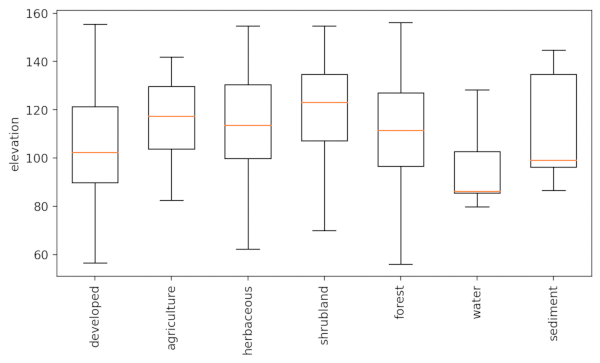### Example 3

Draw boxplots of the values of the elevation layer per category from the landclass96 layer from the same NC sample dataset. Set the -o flag to include outliers. Use order=ascending to order the boxplots from low to high median. Provide a name for the outlier map to save the outlier locations as a point vector map.
```r.boxplot -o order=ascending input=elevation zones=landclass96 output="r_boxplot_03.png" map_outliers="outliers"
```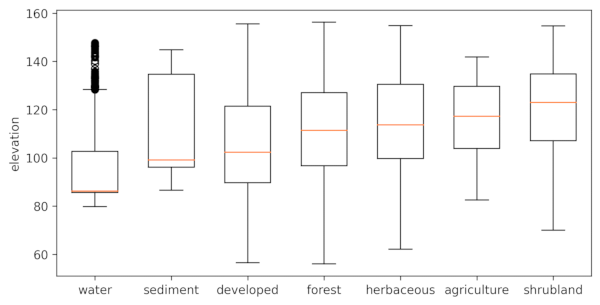Below, part of the landclass96 raster map is shown, with on top the vector point layer with location of outliers. Curiously, for some lakes, only part of the raster cells are outliers.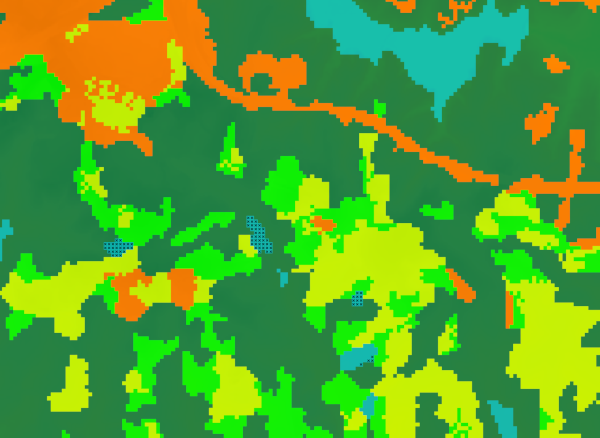### Example 4

Draw boxplots of the values of the elevation layer per category from the landclass96 layer from the same NC sample dataset. Set the -c flag to color the boxplots, use order=ascending to order the boxplots from low to high median, and set the font size to 11.
```r.boxplot -c order=ascending fontsize=11 input=elevation zones=landclass96 output="r_boxplot_04.png"
```

### Example 5

To make it easier to compare the elevation distribution across the different land use categories, you can plot a line and band representing the median and interquartile range (IQR) of the whole raster layer.
```r.boxplot -c input=elevation zones=landclass96 raster_statistics=median,IQR
```

Note, if the zones of your zonal map do not cover the entire area, you may want to use r.mask to mask out the non-covered parts of the input map, or alternatively, create a new input raster with only values within the zones of the zonal layer.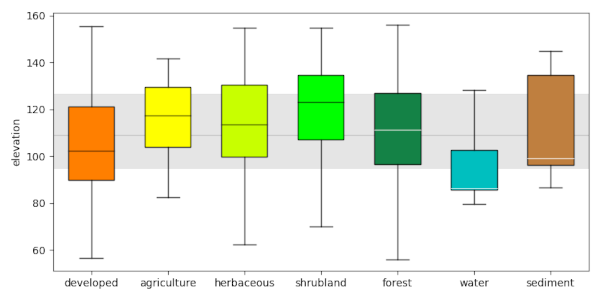## Acknowledgements

This work was carried in the framework of the Save the tiger, save the grassland, save the water project by the Innovative Bio-Monitoring research group.

r.scatterplot, r.stats.zonal, d.vect.colbp

## AUTHOR

Paulo van Breugel
Applied Geo-information Sciences
HAS University of Applied Sciences

## SOURCE CODE

Available at: r.boxplot source code (history)

Latest change: Monday Jan 30 19:52:26 2023 in commit: cac8d9d848299297977d1315b7e90cc3f7698730

© 2003-2023 GRASS Development Team, GRASS GIS 8.2.2dev Reference Manual